Main Content

# Simulate Uncertain Model at Sampled Parameter Values

This example shows how to simulate an uncertain model in Simulink® using the Uncertain State Space block. You can sample uncertain parameters at specified values or generate random samples. The MultiPlot Graph block lets you visualize the responses of multiple samples on the same plot.

### Uncertain Model

The simple model `rctUncertainModel` contains an Uncertain State Space block with a step input. The step response signal feeds a MultiPlot Graph block.

```mdl = "rctUncertainModel"; open_system(mdl) ```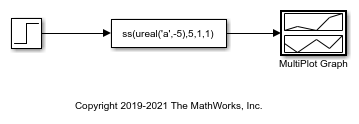By default, the Uncertain State Space block is configured to simulate the uncertain model `ss(ureal('a',-5),5,1,1)`, which is a `uss` model with one uncertain parameter. For this example, create a model of a mass-spring damper system with an uncertain spring constant and damping constant.

```m = 3; c = ureal('c',1,'Percentage',20); k = ureal('k',2,'Percentage',30); usys = tf(1,[m c k]) ```
```usys = Uncertain continuous-time state-space model with 1 outputs, 1 inputs, 2 states. The model uncertainty consists of the following blocks: c: Uncertain real, nominal = 1, variability = [-20,20]%, 1 occurrences k: Uncertain real, nominal = 2, variability = [-30,30]%, 1 occurrences Type "usys.NominalValue" to see the nominal value, "get(usys)" to see all properties, and "usys.Uncertainty" to interact with the uncertain elements. ```

To simulate this system, in the block parameters, enter `usys` for the Uncertain system variable parameter.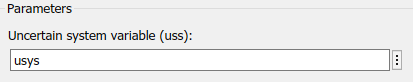Alternatively, set the parameter value at the command line.

```ublk = strcat(mdl,"/Uncertain State Space"); set_param(ublk,"USystem","usys"); ```

### Simulate Nominal Model

To simulate the model, Simulink must set the uncertain parameters in `usys` to specific, non-uncertain values. Use the Uncertainty value parameter to specify these values. By default, this parameter is set to `[]`, which causes Simulink to use the nominal values of all uncertain parameters.

Simulate the model. The MultiPlot Graph block generates a plot of the nominal model response to the step input signal.

```sim(mdl); ```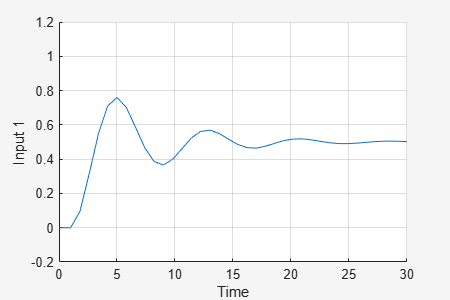### Simulate Specified Samples

To simulate the uncertain model with the uncertain parameters set to values other than the nominal values, set the Uncertainty value parameter to a structure whose fields are the uncertain elements of the `uss` model. For instance, create a structure `samps` that sets the damping constant to 1.2 and the spring constant to 1.7.

```samps = struct('c',1.2,'k',1.7); ```

Set the Uncertainty value parameter to `samps`, and simulate the model. The MultiPlot Graph block adds this new system response to the same axis as the previous response.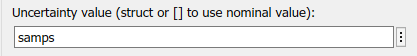```set_param(ublk,"UValue","samps"); sim(mdl); ```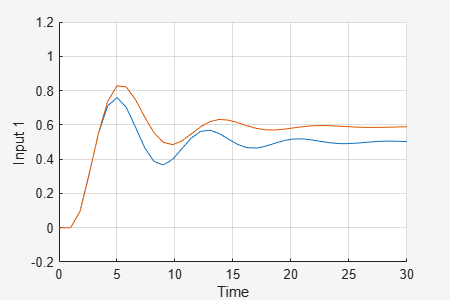### Simulate Random Samples

You can use the `usample` command to generate samples of `usys` at random values of the uncertain parameters. The command `uvars = ufind(mdl)` generates a structure containing all the uncertain parameters in the model.

```uvars = ufind(mdl); ```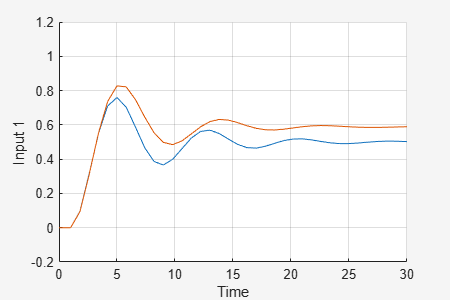`usample` takes random samples of these parameters and returns a structure you can use for the Uncertainty value parameter. Set Uncertainty value to `usample(uvars)`, and simulate the model.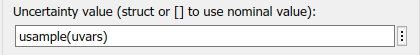```set_param(ublk,"UValue","usample(uvars)"); sim(mdl); ```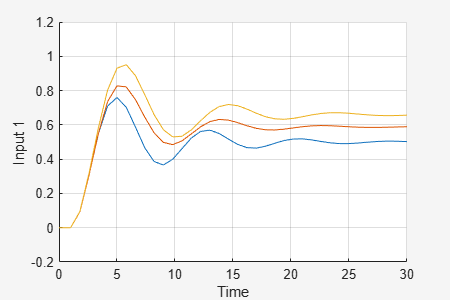The step response of the randomly sampled instance of `usys` is added to the MultiPlot Graph block. Simulate the model ten more times. Each time, `usample` generates new values for `c` and `k`, and the plot is updated with another step response.

```for i=1:10 sim(mdl); end ```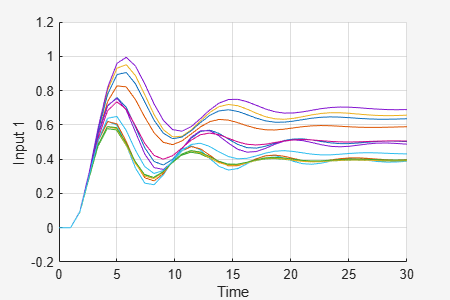## SupportGet trial now